# AP Board 4th Class Maths Solutions 8th Lesson Data Handling

Andhra Pradesh AP Board 4th Class Maths Solutions 8th Lesson Data Handling Textbook Exercise Questions and Answers.

## AP State Syllabus 4th Class Maths Solutions Chapter 8 Data Handling

Textbook Page No. 105

Do this

Observe the two tables and write the number for the given tally marks, and tally marks for the numbers.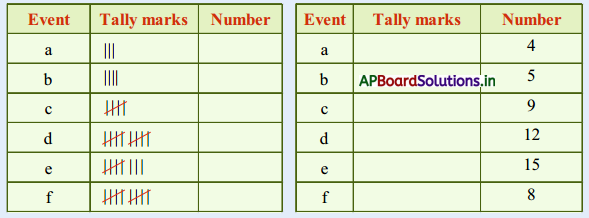Solution: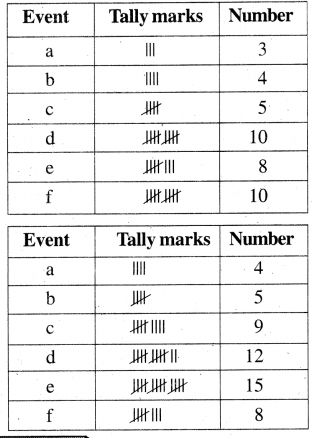Try this

Balu has saved ₹ 1, ₹ 2 and ₹ 5 coins in his kiddy bank. Now his kiddy bank is full and he wanted to count the number of each coin separately. How will he do this in an easy way ? He can do this in making following table.
Solution: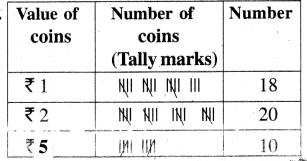Textbook Page No. 107

Do this

Collect the necessary information to find the most favoured flower / vegetable of your class. Represent the data in a) tabular form b) pictograph.

a) Tabular form:
Solution: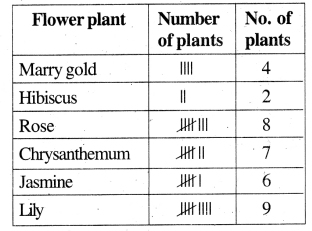b) pictograph :
Solution: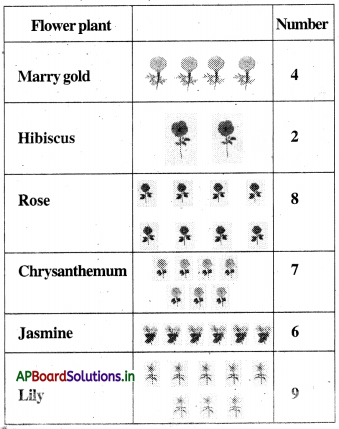Vegetables :

a) Tabular form: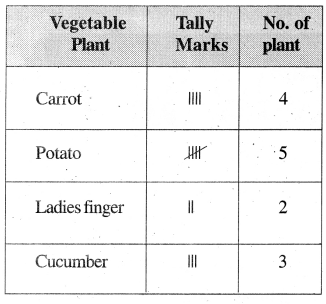b) Pictograph: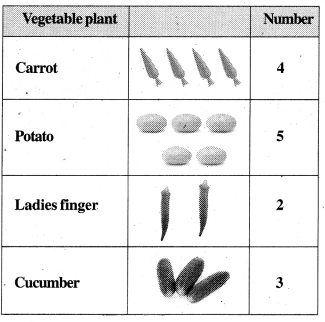Textbook Page No. 108

Do this

The following pictograph depicts the types of household in a street of Unnava village. Observe the table and complete it.

In Unnava village three types of houses are there in street. Number of houses of each type is in the table given below. Fill in the table using tally marks.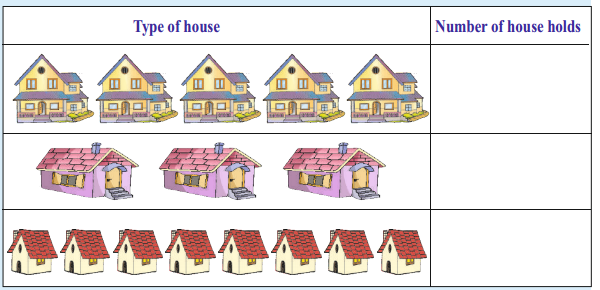Solution: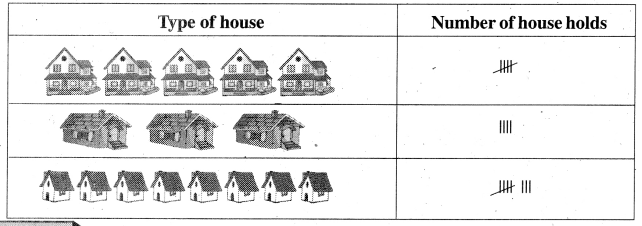Try this

Below is a pictograph showing the number of students of a class who liked different food items.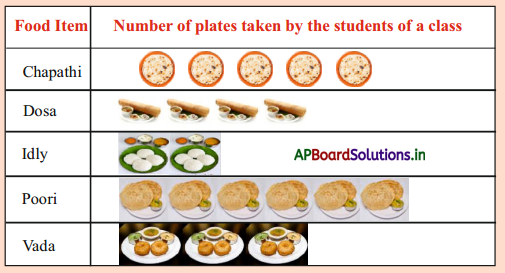By Observing the above pictograph answer the following questions.
i) How many students like dosa ?
Solution:
4 members liked dosa.

ii) Which food item is the least liked ? How many students like it ?
Solution:
Idly is the least liked. 2 members liked Idly.

iii) How many students are there in the class ?
Solution:
20 members are there in the class.iv) Which food item is the most liked ? How many students like ?
Solution:
Poori is the most liked. 6 members likes poori.

Textbook Page No. 111

The bar graph shows number of flower plants in the garden of M.P.P. School, Kothapalem.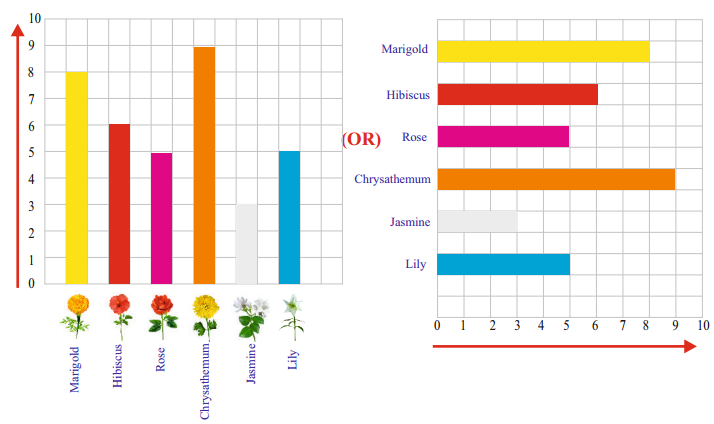Answer the following from the above bar graph.
Question 1.
Number of jasmine plants = _________ + ____________
= 3 + 3 = 6 plants

Question 2.
Number of lily plants = ____________
Solution: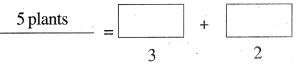Question 3.
Number of marigold plants = ____________
Solution: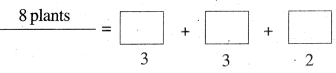Textbook Page No. 112

Do this

The bar graph shows how Kishore spends his time on a normal day.
Scale 1 box = 1 hour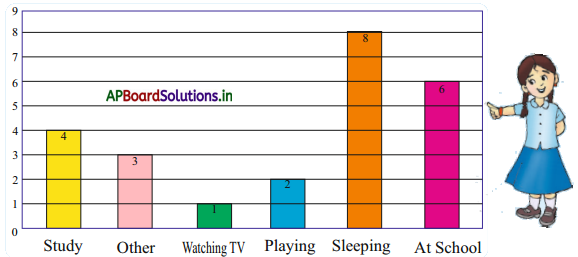i) On which activity does Kishore spend more number of hours ?
Solution:
Kishore spent more number of hours on sleeping.

ii) How many hours does Kishore spend in sleeping and at school ?
Solution:
Kishore spent 8 hours in sleeping and 6 hours at school to tally 14 hours.

iii) On which activity does Kishore spend the least number of hours ?
Solution:
Kishore spent the least number of hours in watching.iv) What is the difference between the number of hours spent in sleeping and the number of hours spent at school ?
Solution:
Difference between both = 8 – 6 = 2 hours.

Exercise – 8.1

Question 1.
30 children of class 4, expressed their pets in their homes as follows…………..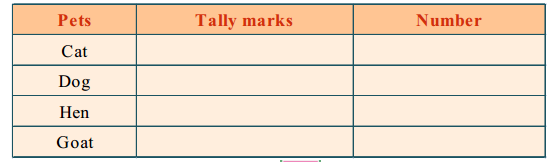Complete the table and answer the questions given below.
Solution: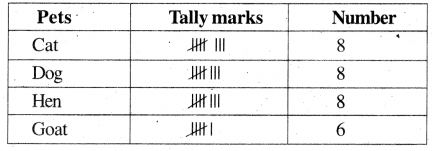a) Which is the most favourite pet ?
Solution:
Cat, Dog and Hen are the most favourite pets.

b) How many children have hen as pet ?
Solution:
8 children have hen as pet.c) How many children have dog as pet ?
Solution:
8 children have dog as pet.

Question 2.
The students have recorded the homes of the little creatures near their houses in the activity book.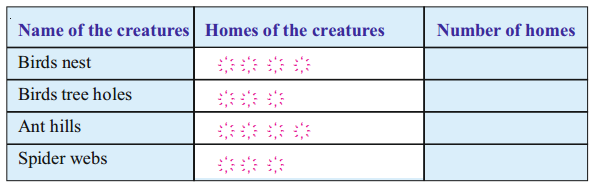Solution: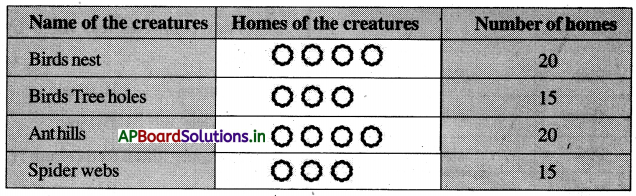a) How many homes have they recorded in their activity book ?
Solution:
70 homes are recorded in their activity book.

b) Which kind of creature homes are same in number and how many ?
Solution:
Tree holes and spider webs are same in number and 15 more.

c) Which kind of creature homes are more than the others ?
Solution:
Birds nest and Ant hills are more than the others.Question 3.
Observe the bar graph and answer the following. The bar graph shows the number of varieties of flowers used to make bouquets in a day by a flower vendor.
Scale : 1 box = 10 flowers.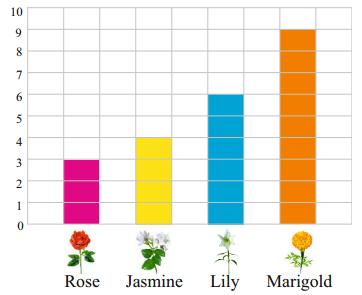a) How many roses are used in a day?
Solution:
3 boxes = 3 × 10
= 30 flowers are used in a day.

b) Which flower is used the least ?
Solution:
Rose flower is used the least.

c) How many flowers are used totally?
Solution:
Totally used flowers are
9 + 6 + 4 + 2 = 22 boxes × 10
= 220 flowersd) Which flower is used the least ?
Solution:
Marigold is used the most.

Multiple Choice Questions

By using the given data, choose correct option in the questions given below.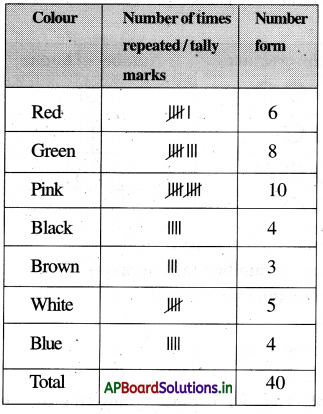Question 1.
What colour is mostly liked by the class ?
A) Green
B) Pink
C) Red
D) White
B) Pink

Question 2.
What colour is less liked by the class ?
A) Brown
B) Black
C) White
D) Pink
A) BrownQuestion 3.
How many liked Red colour ?
A) 6
B) 8
C) 10
D) 5
A) 6

Question 4.
What is the difference between numbers who liked pink and white ?
A) 6
B) 4
C) 5
D) 3
C) 5

By using the given data, choose correct option in the questions given below.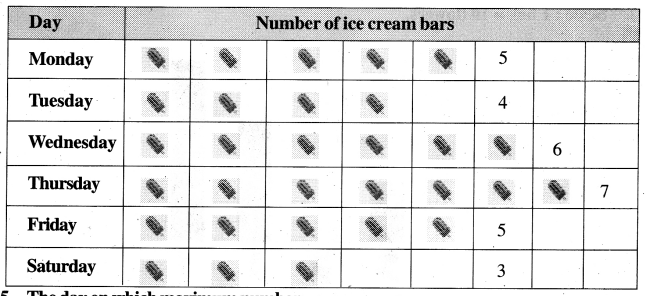Question 5.
The day on which maximum number of ice cream bars were sold is
A) Thursday
B) Wednesday
C) Friday
D) Saturday
A) ThursdayQuestion 6.
The day on which minimum number of ice cream bars were sold is
A) Thursday
B) Wednesday
C) Friday
D) Saturday
D) Saturday

Question 7.
Total number of ice-cream bars sold during the week
A) 30
B) 20
C) 40
D) 50